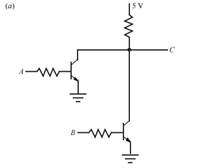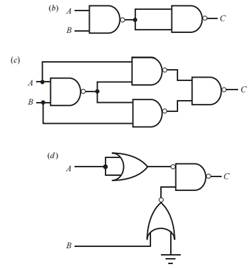Create an Account

Home / Questions / Write out a simplified Boolean expression and construct a truth table for each of the foll...

Write out a simplified Boolean expression and construct a truth table for each of the following circuits Assume

Write out a simplified Boolean expression and construct a truth table for each of the following circuits. Assume 0 = low = 0 V and 1 = high = 5 V.Hint: Try to write out a quasi-logic statements (see Sections 6.6.1 and 6.6.2 ) or a truth table first, based on how the transistors function. Assume ideal transistors operating either in cutoff or saturation.Jul 27 2020 View more View LessSubscribe To Get Solution